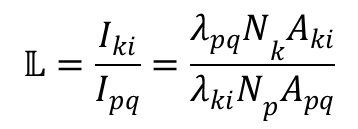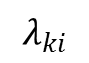### Line Intensity Ratio

In optical plasma diagnostics, line intensity ratio method is one of method to determine electron temperature and density of plasma. The intensity of radiation, Iki, is expressed byEki : Difference between energy levels Ek and Ei (Ek>Ei)
Aki : Transition probability
Nk : Population of upper energy state of k.

Line intensity ratio of plasma is expressed by: Wavelength corresponding to the transition from energy state k to energy state i.

In experiment, the optical system cannot collect whole radiation from plasma volume, the transmittance of optical system has a dependency on wavelength, and quantum efficiency of detecting system is also dependent on a wavelength. Thus, the measured line intensity ratio needs to be relatively calibrated for whole optical system and detector.